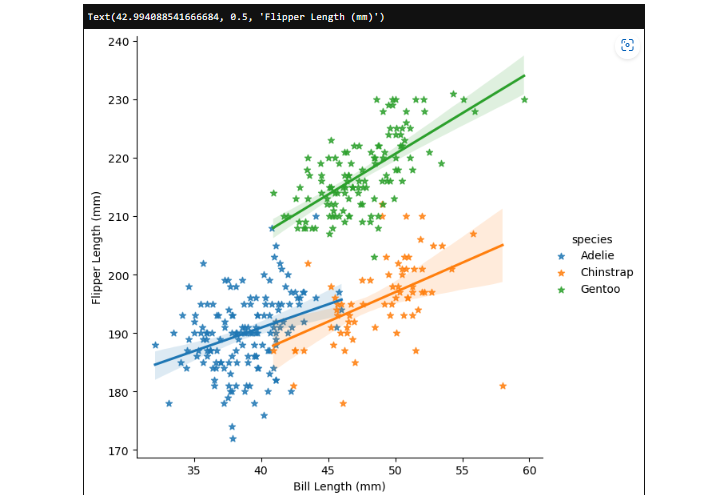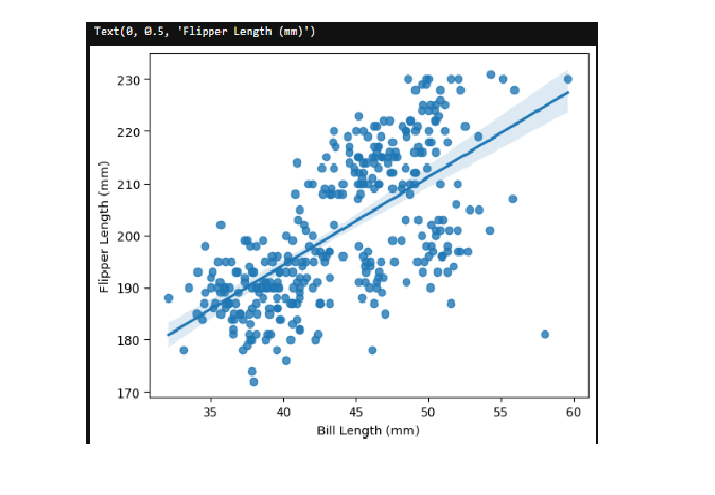# How to add Regression Line Per Group with Seaborn in Python?

One of the most useful tools provided by Seaborn is the ability to add regression lines to a scatterplot. Regression lines can be helpful in analyzing the relationship between two variables and identifying trends in the data.

In this article, we will learn how to add Regression Line Per Group with Seaborn in Python. Seaborn has more than one way to make scatter plots between two numbers. For example, to make the plot we need, we can use the lmplot() function.

## Seaborn

Seaborn is a library of Python for making graphs based on statistics. It is built on top of matplotlib and works efficiently with the data structures of Pandas. Seaborn helps you look at your data and figure out what it means. Its plotting functions work on arrays and data frames that contain whole datasets and do the statistical aggregation and semantic mapping needed to make plots that are useful.

Its declarative API is based on datasets, so you can focus on what the different parts of your plots mean instead of how to draw them. Seaborn seeks to make visualizing data the primary method of looking at and understanding it. It gives us APIs that are focused on datasets, so we can switch between different ways of seeing the same variables to better understand the dataset.

## Regression Line

A regression line is a line that shows how a set of data changes over time. In other words, it shows the best trend from the data that has been given.

Regression lines are helpful when making predictions. Its goal is to explain how the dependent variable (the y variable) is related to one or more independent variables (x variable).

If we put different values for the variables that are independent into the equation we get from the regression line, we can predict how the dependent variables will behave in the future. This type of line is mainly used with scatter plots.

## Scatter plot

Scatterplot is used to group items by significance, which can help you understand them better in a graph. They can make two-dimensional graphics that can be improved by mapping up to three more variables while using the meanings of hue, size, and style parameters. All of the parameters control the visual and semantic information that is used to tell the different subsets apart. It can help to use redundant semantics to make graphs easier to understand.

## Scatter plot and regression line

The scatterplot compares the values of one variable to the values of another variable. Seeing the pattern or how close the points are to each other helps us figure out how the two variables are related. On the other hand, the regression line only connects the variables that are already being studied if they seem to have a strong link. The scatterplot can give you an idea of the relationship, but to be sure, we can also do a hypothesis test. The scatterplot and regression line can be used to find out if any of the (x,y) pairs are outliers, to predict y at a specific value of x, and to estimate the average y at a specific value of x.

What it doesn't tell us is how x and y are related to each other. The underlying relationship between x and y may or may not be a cause-and-effect relationship, and correlation does not in any way mean that there is a cause-and-effect relationship.

## Adding regression line per group with Seaborn

Seaborn has more than one way to make scatter plots between two numbers. We can make a scatter plot with Seaborn by using the lmplot(), regplot(), and scatterplot() functions. But they are not the same in how they can add a regression line to the scatter plot.

First, we'll look at two ways to add a simple regression line to a scatter plot in Seaborn. To add a single regression line, we will use the lmplot() and regplot() functions. When you have a set of data with a third categorical variable, it can be helpful to add a regression line for each group.

### Adding regression line per group with Seaborn using lmplot()

In a scatterplot, we will use the lmplot() function to add a regression line for each group.

### Example

import seaborn

# use lmplot
import matplotlib.pyplot as pltt
seaborn.lmplot(x="bill_length_mm",
y="flipper_length_mm",
hue="species",
markers='*',
data=pg,
height=6)

pltt.xlabel("Bill Length (mm)")
pltt.ylabel("Flipper Length (mm)")


### Output### Adding regression line per group with Seaborn using regplot()

In a scatterplot, we will use the regplot() function to add a regression line for each group.

### Example

import seaborn
# use lmplot
import matplotlib.pyplot as pltt
seaborn.regplot(x="bill_length_mm",
y="flipper_length_mm",
data=pg,
)
pltt.xlabel("Bill Length (mm)")
pltt.ylabel("Flipper Length (mm)")


### Output## Conclusion

In this article, we learned that Regression lines are used to make predictions by using the x and y variables. We understood that regression lines are mainly used with scatter plots using Seaborn library of Python. We also discovered that there are mainly two methods in the seaborn using which we can add regression lines to a scatter plot they are regplot() and lmplot().

Updated on: 31-May-2023

220 Views